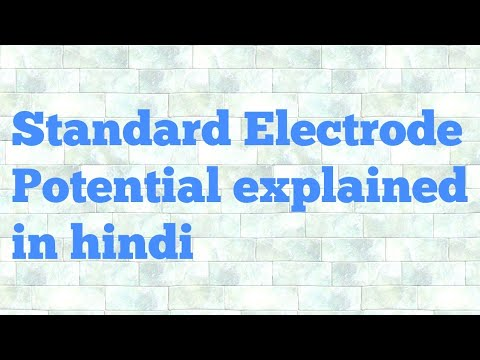Courses

# Test: Standard Electrode Potential

## 10 Questions MCQ Test Chemistry for JEE | Test: Standard Electrode Potential

Description
This mock test of Test: Standard Electrode Potential for JEE helps you for every JEE entrance exam. This contains 10 Multiple Choice Questions for JEE Test: Standard Electrode Potential (mcq) to study with solutions a complete question bank. The solved questions answers in this Test: Standard Electrode Potential quiz give you a good mix of easy questions and tough questions. JEE students definitely take this Test: Standard Electrode Potential exercise for a better result in the exam. You can find other Test: Standard Electrode Potential extra questions, long questions & short questions for JEE on EduRev as well by searching above.
QUESTION: 1

### Chemical used in salt bridge is:

Solution:

KCl is used as salt bridge because it provides positive K+ ions and negative Cl- ions as the salt bridge needs to maintain the neutrality in the system by providing enough negative ions equal to the positive ions during oxidation.

QUESTION: 2

### Hydrogen gas is not liberated when the following metal is added to dil. HCl.

Solution:

The metals, present below hydrogen in the electrochemical series, cannot liberate hydrogen from the dilute acids.
Among the given metals only Ag is present below hydrogen in electrochemical series, so it does not evolve hydrogen with dil HCl.
Ag−IdilHCl ⟶ No reaction

QUESTION: 3

### Temperature for the measurement of standard electrode potential is:

Solution:

The standard electrode potentials are customarily determined at solute concentrations of 1 Molar, gas pressures of 1 atmosphere, and a standard temperature which is usually 25°C i.e, 298 K.

QUESTION: 4

The electrode potential measures the ______.

Solution:
• The tendency of an electrode to lose or gain electrons when it is in contact with its own ions in solution is called electrode potential.
• Since the tendency to lose electrons means also the tendency to get oxidised, this tendency is called oxidation potential.
• Similarly, the tendency to gain electrons means the tendency to get reduced.
• Hence this tendency is called reduction potential.
QUESTION: 5

The reduction potential of an element A is 1.71 V. What can be concluded from this?

Solution:
• The standard reduction potential is the tendency for a chemical species to be reduced, and is measured in volts at standard conditions.
• The more positive the potential is the more likely it will be reduced. Hence, A will undergo reduction easily.
QUESTION: 6

Stronger the oxidizing agent, greater is the :

Solution:

Stronger oxidizing agent means more easily it undergoes reduction and thus have greater standard reduction potential.
Remember, Li is the strongest reducing agent and F is the strongest oxidizing agent

QUESTION: 7

Consider the following reaction which of the following statement is true for this cell reaction.
(Zn + Cu2+ → Zn2+ + Cu)

Solution:
• For the reaction Zn + Cu2+ → Zn2+ + Cu, Zn is oxidized to Zn2+ while Cu2+ is reduced to Cu
• In a redox reaction, the reactant that loses electrons (is oxidized) causes a reduction and is called a reducing agent. In the example above, zinc metal is the reducing agent; it loses two electrons (is oxidized) and becomes Zn2+ ion.
QUESTION: 8

A half cell reaction A- → A + e- has a large negative reduction potential. It follows that :

Solution:

Large negative RP or more positive oxidation potential and thus, more is the tendency to get oxidized.

QUESTION: 9

Two elements A and B have reduction potential’s 0.23V and 0.46V which statement is true regarding these two elements.

Solution:

The more positive the reduction potential value, the more is the tendency of the element to reduce itself and hence more will be the tendency to accept electrons.

Hence the other element will have more tendency to lose electrons

QUESTION: 10

The reduction potential of an element A is -2.71V. What can be concluded from this?

Solution:

Reduction potential means to accept electrons to reduce oneself.
A + e- → A- ∆Ereduction = +ve value
Since, the reduction potential is negative, it means that the reaction will reverse to make ∆E value +ve. So the reaction becomes,
A → A+ + e-
This becomes oxidation of A. So oxidation of A will be easy.

Track your progress, build streaks, highlight & save important lessons and more!

### Similar Content### Related tests